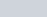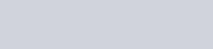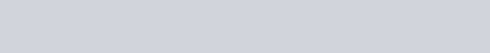1. Home
2. Docs
3. Numerical Methods
4. Interpolation and Regression

# Interpolation and RegressionGiven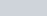data points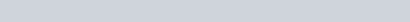interpolation is the process of finding an equation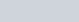that passes through abovedata points and using this equation to find value of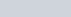.

## Errors in Polynomials

(eq 1)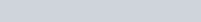Let us consider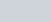=>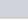degree polynomial through thepoints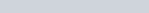Since both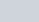andhave the same value at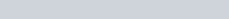using eq 1

(eq 2)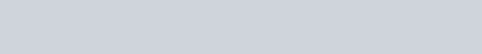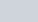represting error at the non-tabulated points

using eq 1

(eq 3)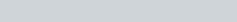assigning eq 2 in eq 3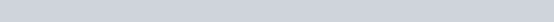let us define a function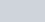, which is the function ofand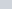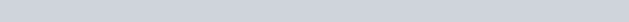at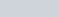where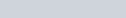is zero and also at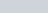,
There are about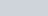zero’s atIf we impose the law of mean value on, thenmust be continuous and differentiable. Also there exists a root ofbetween each of the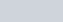zeros ofthat is there and a total ofzeros of. If there exists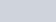then there arezeros and similarly if thederivative exists then there exist at least a zero in the interval of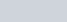let us call this as